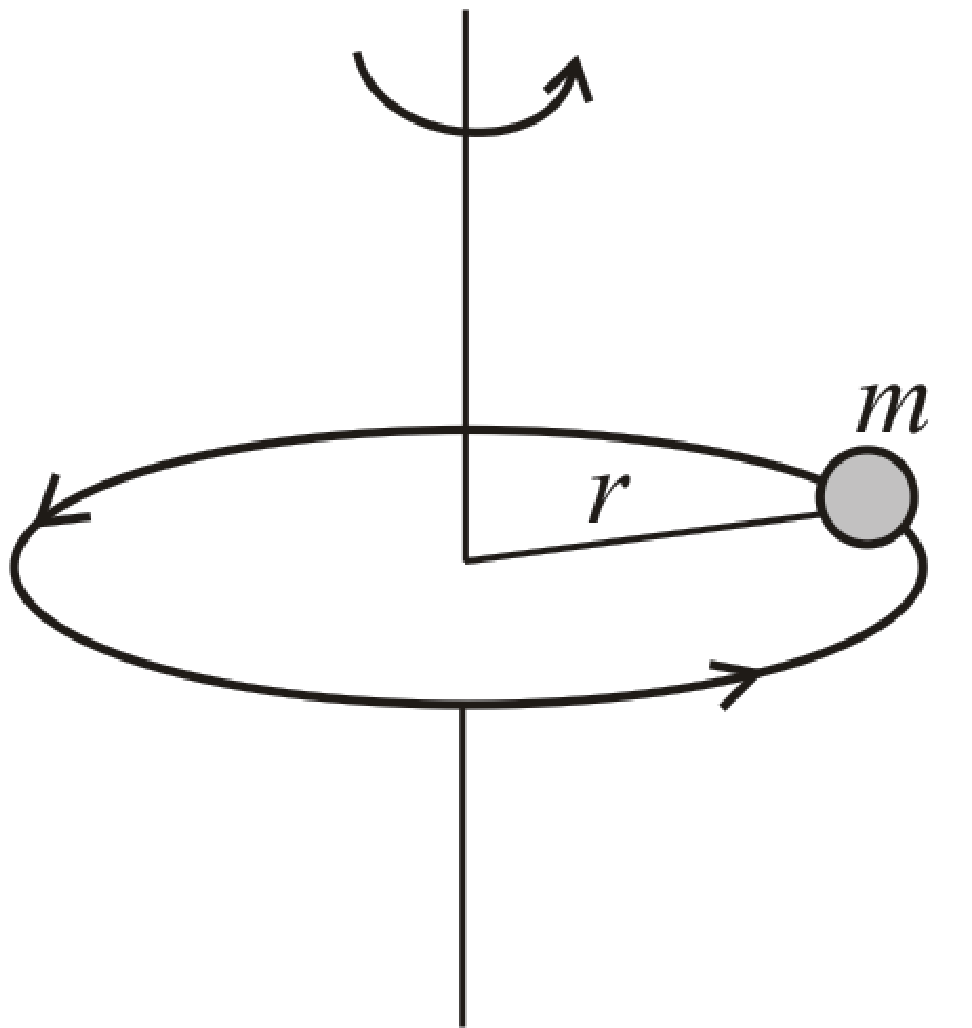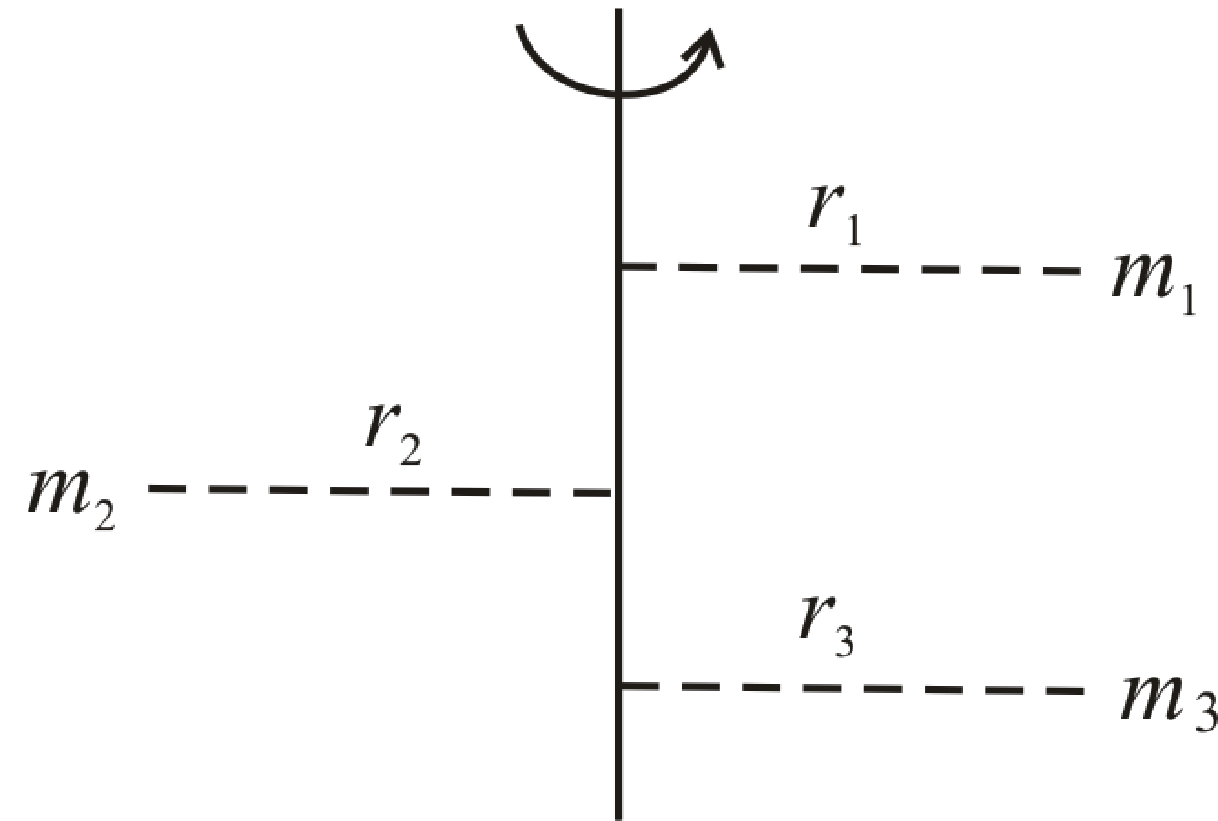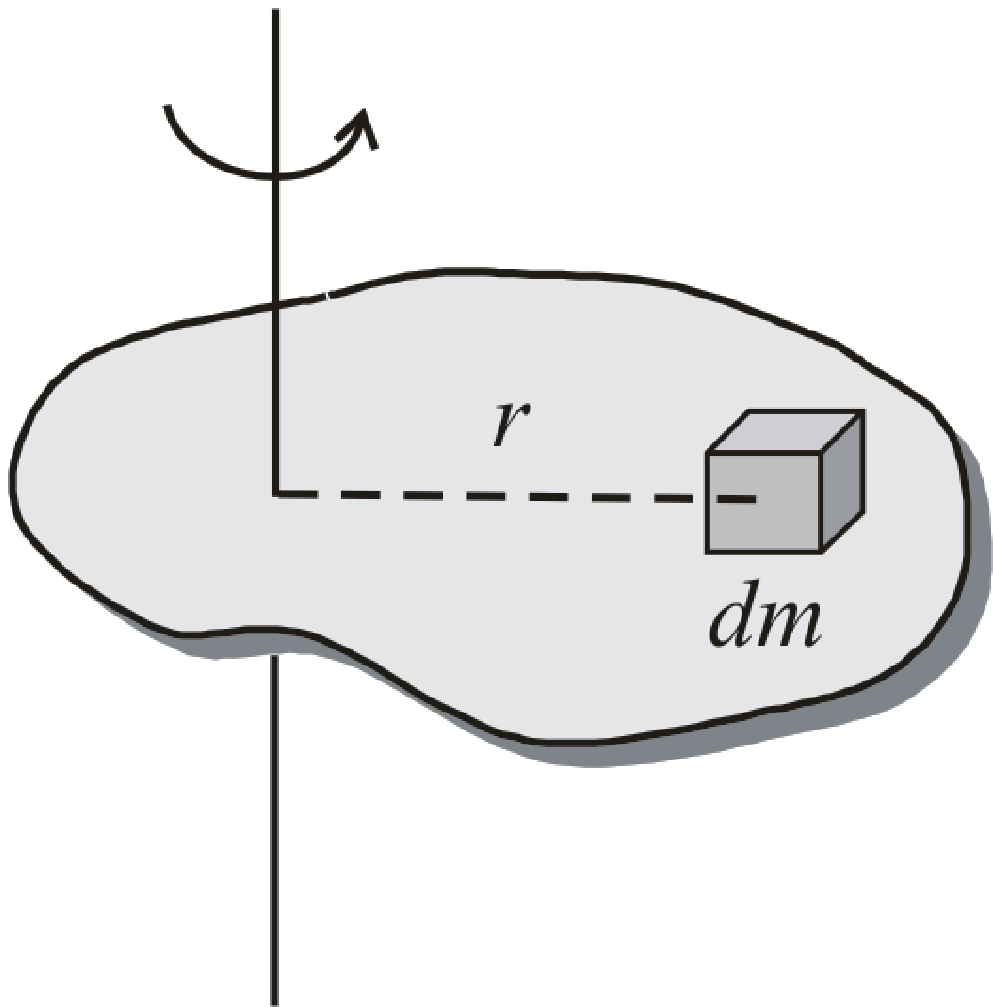BodhiAI
Oct. 31, 2019

#### Moment of Inertia:

Moment of Inertia:

(i) Moment of inertia of a particle  I = mr2; where r  is the perpendicular distance of particle from rotational axis.

(ii) Moment of inertia of a body made up of number of particles (discrete distribution)(iii) Moment of inertia of a continuous distribution of mass, treating the element of mass dm at positionr as particle

dI = dmr2 i.e.(iv) Dimension : [ML2T0]

(v) S. I. Unit :kg-m2

(vi) Moment of inertia depends on mass, distribution of mass and on the position of axis of rotation.

(vii) Moment of inertia does not depend on angular velocity, angular acceleration, torque, angular momentum and rotational kinetic energy.